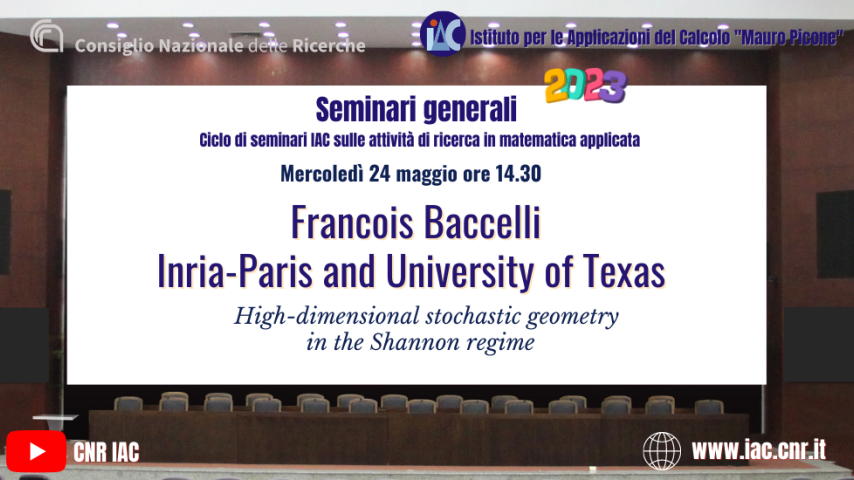# FRANCOIS BACCELLI PER I SEMINARI GENERALI DELL'IACMercoledì 24 maggio un nuovo seminario della serie "Seminari generali dell'IAC 2023".

Ospite è Francois Baccelli, Direttore di ricerca presso Inria Paris research centre e capo del Simons Center on Communication, Information and Network Mathematics all'Università del Texas.

Titolo del seminario: High-dimensional stochastic geometry in the Shannon regime.

Abstract: This talk will focus on Euclidean stochastic geometry in the Shannon regime. In this regime, the dimension n of space tends to infinity, point processes have intensities which are exponential functions of n, and the random compact sets have diameters of order square root of n.
Three basic models of stochastic geometry based on Poisson processes will be considered: the Boolean model, the Poisson-Voronoi tessellation and the Poisson hyperplane tessellation.
We will show how to calculate the asymptotic behavior of the classical quantities of stochastic geometry for these three models in the Shannon regime: for the Boolean model, the volume fraction, the percolation threshold or the size of the connected components; for tessellations, statistics of geometric properties of cells (volume, diameter, etc.).
These questions are motivated by problems in information theory, including the calculation of error exponents for channel coding based on random codes and calculating the distortion in the one-bit compression-based source coding.

Survey of work in collaboration with Venkat Anantharam (UC Berkeley) and Eliza O'Reilly (Caltech).

Contatti (IAC)
Data inizio